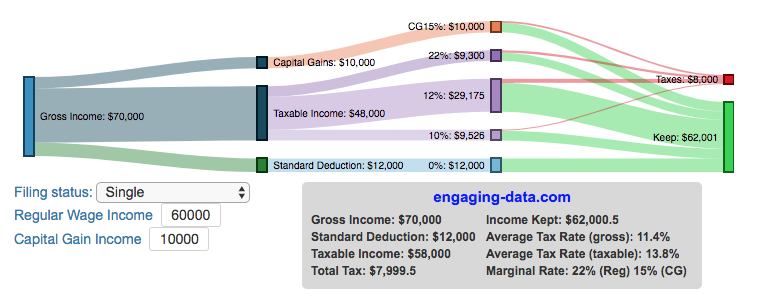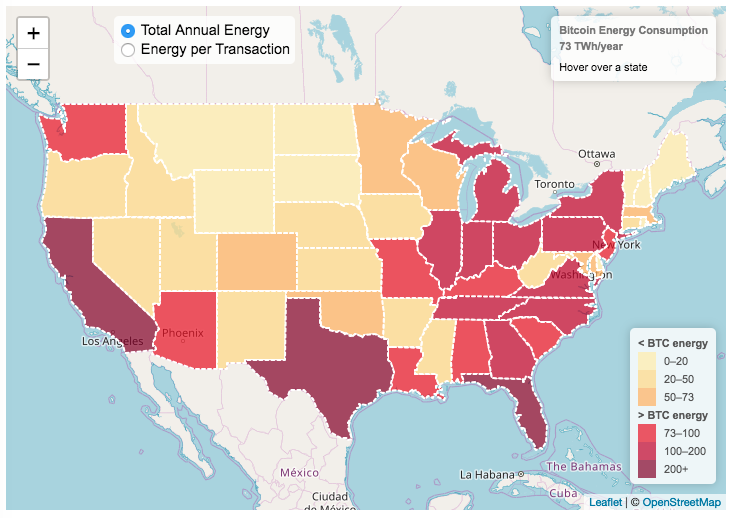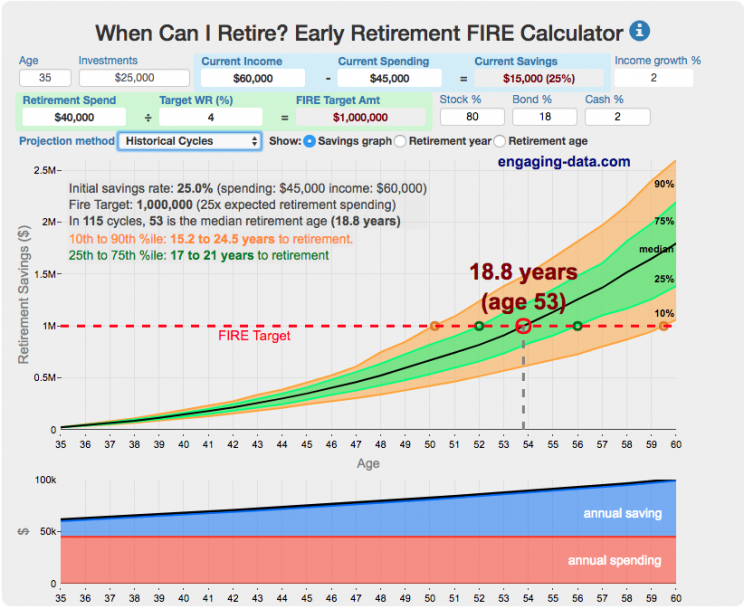# Archive for the ‘Money’ Category:

### Understanding Tax Brackets: Interactive Income Tax Visualization

Posted In: Money

##### How is your income distributed across tax brackets?

I previously made a graphical visualization of income and marginal tax rates. That graph tried to show alot of info on the same graph, i.e. the breakdown of income tax brackets for incomes ranging from $10,000 to$3,000,000. It was nice looking, but I think several people were confused about how to read the graph. This Sankey graph is a more detailed look at the tax breakdown for one specific income. You can enter your (or any other) profile and see how taxes are distributed across the different brackets. It can help (as the other tried) to better understand marginal and average tax rates. This tool only looks at US Federal Income taxes and ignores state, local and Social Security/Medicare taxes.

– Use this button to generate a URL that you can share a specific set of inputs and graphs. Just copy the URL in the address bar at the top of your browser (after pressing the button).

Instructions for using the visual tax calculator:

• Select filing status: Single, Married Filing Jointly or Head of Household. For more info on these filing categories see the IRS website
• Enter your regular income and capital gains income. Regular income is wage or employment income and is taxed at a higher rate than capital gains income. Capital gains income is typically investment income from the sale of stocks or dividends and taxed at a lower rate than regular income.
• Move your cursor or click on the Sankey graph to select a specific link. This will give you more information about how income in a specific tax bracket is being taxed.
• #### **Click Here to view other financial-related tools and data visualizations from engaging-data**

As seen with the marginal rates graph, there is a big difference in how regular income and capital gains are taxed. Capital gains are taxed at a lower rate and generally have larger bracket sizes. Generally, wealthier households earn a greater fraction of their income from capital gains and as a result of the lower tax rates on capital gains, these household pay a lower effective tax rate than those making an order of magnitude less in overall income.

Here are two tables that lists the marginal tax brackets in the United States in 2018 that form the basis of the calculations in the calculator. 2019’s numbers are pretty similar.

US Tax Brackets and Rates for 2018
Rate Single
Taxable Income Over
Married Filing Joint
Taxable Income Over
Taxable Income Over
10% $0$0 $0 12%$9,525 $19,050$13,600
22% $38,700$77,400 $51,800 24%$82,500 $165,000$82,500
32% $157,500$315,000 $157,500 35%$200,000 $400,000$200,000
37% $500,000$600,000 $500,000 You can see that tax rates are much lower for capital gains in the table below than for regular income (table above). Capital Gains Brackets for 2018 Single Capital Gains Over Married Filing Jointly Capital Gains Over Heads of Households Capital Gains Over 0%$0 $0$0
15% $38,700$77,400 $51,850 20%$426,700 $480,050$453,350

For those not visually inclined, here is a written description of how to apply marginal tax rates. The first thing to note is that the income shown here in the graphs is taxable income, which simply speaking is your gross income with deductions removed. The standard deduction for 2018 range from $12,000 for Single filers to$24,000 for Married filers.

• If you are single, all of your regular taxable income between 0 and $9,525 is taxed at a 10% rate. This means that your all of your gross income below$12,000 is not taxed and your gross income between $12,000 and$21,525 is taxed at 10%.
• If you have more income, you move up a marginal tax bracket. Any taxable income in excess of $9,525 but below$38,700 will be taxed at the 12% rate. It is important to note that not all of your income is taxed at the marginal rate, just the income between these amounts.
• Income between $38,700 and$82,500 is taxed at 24% and so on until you have income over $500,000 and are in the 37% marginal tax rate . . . • Thus, different parts of your income are taxed at different rates and you can calculate an average or effective rate (which is shown in the summary table). • Capital gains income complicates things slightly as it is taxed after regular income. Thus any amount of capital gains taxes you make are taxed at a rate that corresponds to starting after you regular income. If you made$100,000 in regular income, and only $100 in capital gains income, that$100 dollars would be taxed at the 15% rate and not at the 0% rate, because the $100,000 in regular income pushes you into the 2nd marginal tax bracket for capital gains (between$38,700 and $426,700). Data and Tools: Tax brackets and rates were obtained from the IRS website and calculations were made using javascript and code modified from the Sankeymatic plotting website.### Visual Guide to Understanding Marginal Tax Rates Posted In: Money ##### What is a marginal tax rate? There is a fair amount of confusion about what a marginal tax rate is and how it affects how much tax you would owe the government on a certain amount of income. These graphs are here to help you better understand the difference between a marginal and average tax rate and to easily calculate these rates for specific examples in the US context. This tool only looks at US Federal Income taxes and ignores state, local and Social Security/Medicare taxes. Marginal tax rates are the rate at which an additional dollar of income will be taxed at. There are different tax brackets (each with its own marginal rate) depending on which dollar of income you are looking at. This is very different from the Average (or effective) tax rate that is the result of applying these marginal tax rates across all of your income. #### **Click Here to view other financial-related tools and data visualizations from engaging-data** Instructions for using the visual tax calculator: • Select filing status: Single, Married Filing Jointly or Head of Household. For more info on these filing categories see the IRS website • Select percentage of regular income vs capital gains income. Regular income is wage or employment income and is taxed at a higher rate than capital gains income. Capital gains income is typically investment income from the sale of stocks or dividends and taxed at a lower rate than regular income. • Move your cursor or click on the graph to select a specific income Make sure you note that the x-axis is a logarithmic-scale, meaning that income grows exponentially as you move to the right. • Choose your graph preference One graph (Individual Tax Brackets) shows the individual tax brackets and how much of your income is taxed at the different marginal rates. The other graph (Aggregate Rates) shows the net result of applying the different rates to get your effective rate. One of the most interesting things is to vary the proportion of regular income vs capital gains taxes. Generally, wealthier households earn a greater fraction of their income from capital gains and as a result of the lower tax rates on capital gains, these household pay a lower effective tax rate than those making an order of magnitude less in overall income. Here are two tables that lists the marginal tax brackets in the United States in 2019 that form the basis of the calculations in the calculator. 2018’s numbers are pretty similar. US Tax Brackets and Rates for 2019 Rate Single Taxable Income Over Married Filing Joint Taxable Income Over Heads of Households Taxable Income Over 10%$0 $0$0
12% $9,700$19,400 $13,850 22%$39,475 $78,950$52,850
24% $84,200$168,400 $84,200 32%$160,725 $321,450$160,700
35% $204,100$408,200 $204,100 37%$510,300 $612,350$510,300

You can see that tax rates are much lower for capital gains in the table below than for regular income (table above).

Capital Gains Brackets for 2019
Single
Capital Gains Over
Married Filing Jointly
Capital Gains Over
Capital Gains Over
0% $0$0 $0 15%$39,375 $78,750$52,750
20% $434,550$488,850 $461,700 For those not visually inclined, here is a written description of how to apply marginal tax rates. The first thing to note is that the income shown here in the graphs is taxable income, which simply speaking is your gross income with deductions removed. The standard deduction for 2019 range from$12,200 for Single filers to $24,400 for Married filers. • If you are single, all of your regular taxable income between 0 and$9,700 is taxed at a 10% rate. This means that your all of your gross income below $12,200 is not taxed and your gross income between$12,200 and $21,900 is taxed at 10%. • If you have more income, you move up a marginal tax bracket. Any taxable income in excess of$9,700 but below $39,475 will be taxed at the 12% rate. It is important to note that not all of your income is taxed at the marginal rate, just the income between these amounts. • Income between$39,475 and $84,200 is taxed at 24% and so on until you have income over$510,300 and are in the 37% marginal tax rate . . .
• Thus, different parts of your income are taxed at different rates and you can calculate an average or effective rate (which is shown in the aggregate rates graph).
• Capital gains income complicates things slightly as it is taxed after regular income. Thus any amount of capital gains taxes you make are taxed at a rate that corresponds to starting after you regular income. If you made $100,000 in regular income, and only$100 in capital gains income, that $100 dollars would be taxed at the 15% rate and not at the 0% rate, because the$100,000 in regular income pushes you into the 2nd marginal tax bracket for capital gains (between $39,375 and$434,550).

Data and Tools:
Tax brackets and rates were obtained from the IRS website and calculations were made using javascript and plotted using the plot.ly open source javascript plotting library.### Bitcoin Energy Consumption – Does It Consume More Electricity Than Your State?

Posted In: Energy | Money

This visualization looks at the staggeringly high energy use of Bitcoin and puts it into context: comparing it to electricity usage of US states. Unfortunately for Bitcoin, high energy usage is an intended feature of the system, rather than an unintended consequence. This is because mining is an increasingly energy intensive process, based upon increasingly computationally intensive calculations that are performed on high powered computers and graphical processing units.

Currently, 28 out of 50 states plus the District of Columbia all have lower electricity consumption than estimated annual bitcoin electricity consumption (~73 TWh per year). These states are highlighted in variations of yellow. This is approximately equal to the average annual electricity usage across all US States. States with higher electricity consumption than bitcoin are highlighted in shades of red.

When dividing the total energy use (73 TWh) by the current number of transactions (93 million), we get an average energy consumption of 783 kWh per transaction. Click on the “Energy per Transaction” button to see this visualization. What’s crazy is that a transaction is simply a transfer of bitcoin between “wallets”, recording the transaction, and a validation of the process. There’s no good reason why verifying digital transactions should take this much energy, except that it was built into the fundamental process of validating and mining bitcoin. 783 kWh is larger than the monthly per capita electricity consumption in 10 US states. It could also drive you and your family over 2000 miles in an electric car (e.g. Tesla Model S).

I’m not expert enough in this area to know how much more energy consumption will rise into the future, but if crypto advocates’ predictions come true and bitcoin is used extensively, millions of transactions will occur per hour instead of per year and the price of bitcoin may rise much higher than it currently is. If the price rises, then miners will be willing to expend more energy to “mine” the more valuable bitcoin. Needless to say, this sounds like a very bad idea from an energy consumption and sustainability standpoint.

Data and Tools:
State energy data comes from the US Department of Energy. Estimates of Bitcoin energy use come from Digiconomist’s Bitcoin Energy Consumption Index. The choropleth map is visualized using javascript and the Leaflet.js library with Open Street Map tiles.### When Can I Retire? Early Retirement Calculator / FIRE Calculator

Posted In: Financial Independence | Money

#### How long do I need to save before I can retire?This early retirement calculator / visualizer is designed to project the number of years until you can retire, based upon a few key inputs such as annual income and spending, income growth rate, expected annual spending in retirement and asset allocation. It is a pre-retirement calculator that is useful before you retire to get a sense of how many years it is likely to take to accumulate enough money to retire. The three primary modes that are available in the early retirement calculator are: (1) constant, single fixed-percentage real return rates, (2) historical series of real returns are applied to account for likely variability in future returns and (3) monte carlo simulation of the variable returns based upon user-specified input parameters.

This interactive calculator was built to let you play with the inputs and help you understand how savings rate and retirement spending strongly determine how long it will take you to save up for retirement. Note: it does not simulate the post-retirement period when you start to draw down your savings. That can be done on this post-retirement calculator (Rich, Broke or Dead) which compares the frequency of various outcomes in retirement (running out of money, ending up with way too much money, and life-expectancy).
(more…)

### Post-Retirement Calculator: Will My Money Survive Early Retirement? Visualizing Longevity Risk

Posted In: Financial Independence | Money

I added a simple spending flexibility parameter that allows you to specify how much you could reduce spending when your portfolio is below a certain threshold (in this case, your original retirement amount (inflation adjusted)).